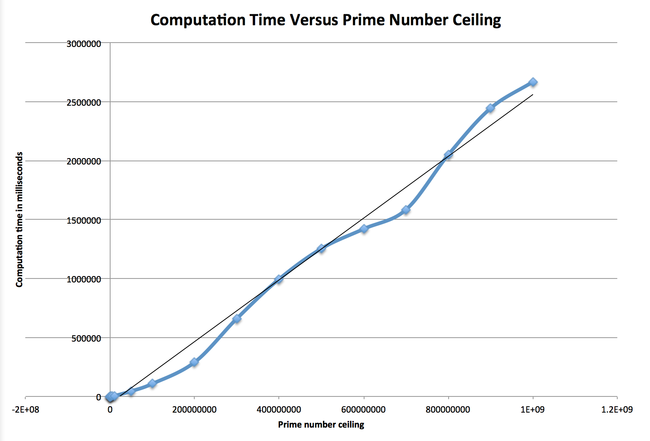# Prime Numbers

Prime numbers are fun and it’s even more fun to come up with ways of finding them. People interested in prime numbers are usually interested in these areas of research:

• Finding algorithms and formulas that yield a lot of prime numbers
• Discovering the largest prime number possible
• Designing algorithms that can evaluate a prime number as fast as possible

In this post I’m just interested in coming up with a very efficient way of finding all the prime numbers up to X. To achieve this, I designed a simple C program that applies a well known algorithm.

# The Algorithm #

First, a prime number is a positive and integer number that can be divided only by itself and 1. Such numbers are:

``````1, 2, 3, 5, 7, 11, 13, 17, 19, 23, 29...
``````

Whether 1 is a prime number or not is up to discussion…

The obvious way to find these numbers is to follow the prime number definition and figure out if each and every number that exists fits. So for every number, find out if it can be divided by anything but itself or one. This is a naive primality test called trial division. To make our algorithm a good one, we want to dismiss a number as quickly as possible so we can move on to the next one.

## Test Only Certain Numbers #

The first clear step is to simply not test even numbers since they all can be divided by 2. The number 2 itself is an exception and its primality is also up to discussion.

## Test Only Certain Factors #

We also want to test as few factors as possible. Therefore it is wise to only try odd numbers in ascending order and stop as soon as it is confirmed as being a factor.

## Test a Subset of Factors #

Now let’s say we’re testing the number 47 to find out whether it’s a prime number or not. Obviously it turns out 47 is indeed a prime number and because of that, we’ll have to test every odd factor from 3 to 45 before we figure out it’s a prime number. Does this sound right? it’s not right because 45 cannot possibly be a factor of 47. So what’s the maximum number that can be a factor of 47? it’s simple it’s the square root of 47 is `6.86`. In short, we only need to test all odd integers that are less or equal to the square root, and that saves a lot of time.

## Test Only Prime Factors #

Currently we’re testing every odd factor. Now these odd factors we’re testing for may be factors of one another. Let’s say we’re testing 83 for primality. The square root of 83 is `9.11`. Therefore we’ll test the factors:

``````3, 5, 7, 9
``````

Do we really need to test 9? 9 is an odd number but it’s also a multiple of 3, which has already been tested. If 83 is divisible by 9 then it has to be divisible by 3. This is why we should only test factors that themselves can’t be decomposed, and those are prime numbers. The efficient way to make this work is to memorize the prime number you have found thus far and re-use that list to test future numbers.

# The C Program #

My program is available at: https://bitbucket.org/tlextrait/prime1
I won’t go over the code, please read the documentation if you are interested.

## Average Times #

Here are stats when running this program on a Macbook Pro running OS X Lion with a 2.53Ghz Intel dual core CPU, 4GB RAM and an SSD.

Average wall times:

• Primes up to 1,000 => 0 milliseconds
• Primes up to 10,000 => 0 milliseconds
• Primes up to 100,000 => 15 milliseconds
• Primes up to 1,000,000 => 240 milliseconds
• Primes up to 10,000,000 => 4.9 seconds
• Primes up to 100,000,000 => 1.5 minute
• Primes up to 1,000,000,000 => 45 minutes

## Trendline #

This numbers above yield the following graph (the Excel file is included with my code on BitBucket).Clearly we have a linear trend, at least up to a billion.